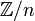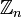# Talk:08-401

something i still unclear on ideal test..

since there are two conditons in ideal test 1. a-b in A whenever a, b in A 2.ra and ar are in A whenever a in A and r in A

i just wonder when i use the ideal test, i need to use both condtions together

or just use one of both , then i can conclude a nonempty subset of a ring R is an ideal of R

thx

I would think both need to be satisfied. Sorin.c

thx so much

now, i have a tiny question, is the Z/n equal to Z_n? are they the same thing.

because the textbook give us the symbol " Z_n", and the lecture notes give us " Z/n"

There is no difference. Dror said he prefers writing$\mathbb Z/n$ instead of$\mathbb Z_n$. Sorin.c